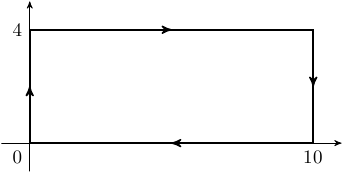# TiKZoriented graph

#### dwsmith

##### Well-known member
How can I draw a rectangle oriented clockwise on the complex plane with vertices on (0,0), (0,4), (10,4), and (10,0)?

I am guessing the tikz package needs to be used but I am not skilled in making pictures.

#### Evgeny.Makarov

##### Well-known member
MHB Math Scholar
If you say \usepackage{tikz}, then you can do

Code:
\tikz\draw (0,0) -- (0,4) -- (10,4) -- (10,0) -- cycle;
or

Code:
\begin{tikzpicture}
\draw (0,0) -- (0,4) -- (10,4) -- (10,0) -- cycle;
\end{tikzpicture}

What do you mean by a rectangle "oriented clockwise"?

I am sure you can easily do this using other LaTeX packages, but I don't know them very well.

•Chris L T521 and dwsmith

#### dwsmith

##### Well-known member
Clockwise is the path you would take around the rectangle.

Ok so that worked but I also want the rectangle on the coordinate axis with arrows along the path and the axes labeled at at 0, 10 and 4. Is there a way to make the rectangle not as big on the pdf?

Last edited:

#### Evgeny.Makarov

##### Well-known member
MHB Math Scholar
Well, after a rectangle is finished being drawn, nobody can tell whether it was drawn clockwise or counterclockwise. So I don't see the meaning in stipulating that it is oriented clockwise.

#### dwsmith

##### Well-known member
Well, after a rectangle is finished being drawn, nobody can tell whether it was drawn clockwise or counterclockwise. So I don't see the meaning in stipulating that it is oriented clockwise.
I need arrows on the rectangle showing its orientation of clockwise.

#### Chris L T521

##### Well-known member
Staff member
If you say \usepackage{tikz}, then you can do

Code:
\tikz\draw (0,0) -- (0,4) -- (10,4) -- (10,0) -- cycle;
or

Code:
\begin{tikzpicture}
\draw (0,0) -- (0,4) -- (10,4) -- (10,0) -- cycle;
\end{tikzpicture}

What do you mean by a rectangle "oriented clockwise"?

I am sure you can easily do this using other LaTeX packages, but I don't know them very well.
Although I've used Tikz for complicated diagrams and images before, I never knew about the cycle option. Thanks for posting that!#### Evgeny.Makarov

##### Well-known member
MHB Math Scholar
Although I've used Tikz for complicated diagrams and images before, I never knew about the cycle option.
Yes, and besides shortening the notation, this option causes TikZ to create a proper join between the first and last segment. Here is a picture from TikZ manual.Here is the code for a rectangle with arrows.

Code:
\usetikzlibrary{arrows}
\begin{tikzpicture}[>=stealth',scale=.5]
\draw[->] (-1,0) -- (11,0);
\draw[->] (0,-1) -- (0,5);
\node[below left] at (0,0) {0};
\node[below] at (10,0) {10};
\node[left] at (0,4) {4};
\draw[thick,->] (0,0) -- (0,2);
\draw[thick,->] (0,2) -- (0,4) -- (5,4);
\draw[thick,->] (5,4) -- (10,4) -- (10,2);
\draw[thick,->] (10,2) -- (10,0) -- (5,0);
\draw[thick] (5,0) -- (0,0);
\end{tikzpicture}
This example uses the scale= option, which can be used in each \draw instruction individually or can apply to the whole picture if specified after \begin{tikzpicture}. It affects the specified coordinates, not the line lengths. The option -> adds the arrow tip only to the end of the path, so the rectangle has to consist of several paths.

A more sophisticated way is to use the decorations library.

Code:
\usetikzlibrary{arrows,decorations.markings}
\begin{tikzpicture}[>=stealth',scale=.5]
\draw[->] (-1,0) -- (11,0);
\draw[->] (0,-1) -- (0,5);
\node[below left] at (0,0) {0};
\node[below] at (10,0) {10};
\node[left] at (0,4) {4};
\draw[
thick,
decoration={
markings,
mark=at position 1/14 with {\arrow{>}},
mark=at position 9/28 with {\arrow{>}},
mark=at position 4/7 with {\arrow{>}},
mark=at position 23/28 with {\arrow{>}}},
postaction={decorate}] (0,0) -- (0,4) -- (10,4) -- (10,0) -- cycle;
\end{tikzpicture}One advantage is that this allows using the cycle construction, which, as said above, create the correct join at (0, 0). I agree that the syntax of the decorations is rather confusing.•dwsmith and Chris L T521

#### dwsmith

##### Well-known member
Yes, and besides shortening the notation, this option causes TikZ to create a proper join between the first and last segment. Here is a picture from TikZ manual.Here is the code for a rectangle with arrows.

Code:
\usetikzlibrary{arrows}
\begin{tikzpicture}[>=stealth',scale=.5]
\draw[->] (-1,0) -- (11,0);
\draw[->] (0,-1) -- (0,5);
\node[below left] at (0,0) {0};
\node[below] at (10,0) {10};
\node[left] at (0,4) {4};
\draw[thick,->] (0,0) -- (0,2);
\draw[thick,->] (0,2) -- (0,4) -- (5,4);
\draw[thick,->] (5,4) -- (10,4) -- (10,2);
\draw[thick,->] (10,2) -- (10,0) -- (5,0);
\draw[thick] (5,0) -- (0,0);
\end{tikzpicture}
This example uses the scale= option, which can be used in each \draw instruction individually or can apply to the whole picture if specified after \begin{tikzpicture}. It affects the specified coordinates, not the line lengths. The option -> adds the arrow tip only to the end of the path, so the rectangle has to consist of several paths.

A more sophisticated way is to use the decorations library.

Code:
\usetikzlibrary{arrows,decorations.markings}
\begin{tikzpicture}[>=stealth',scale=.5]
\draw[->] (-1,0) -- (11,0);
\draw[->] (0,-1) -- (0,5);
\node[below left] at (0,0) {0};
\node[below] at (10,0) {10};
\node[left] at (0,4) {4};
\draw[
thick,
decoration={
markings,
mark=at position 1/14 with {\arrow{>}},
mark=at position 9/28 with {\arrow{>}},
mark=at position 4/7 with {\arrow{>}},
mark=at position 23/28 with {\arrow{>}}},
postaction={decorate}] (0,0) -- (0,4) -- (10,4) -- (10,0) -- cycle;
\end{tikzpicture}One advantage is that this allows using the cycle construction, which, as said above, create the correct join at (0, 0). I agree that the syntax of the decorations is rather confusing.You are great with the tikz stuff. On mhf, you would help with my commutative diagrams. Where do you get a manual for this?

#### Chris L T521

##### Well-known member
Staff member
•dwsmith

#### Evgeny.Makarov

##### Well-known member
MHB Math Scholar
•SammyS, Chris L T521 and dwsmith